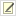# Mutation Rates from Genome Resequencing

Motivation: You have re-sequenced several genomes after a mutation accumulation or adaptive evolution experiment. How do you infer the rates of different types of mutations from these data? What are the 95% confidence intervals on these values?

## Case 1: Mutations with many identical sites

Assumptions:

1. The number of mutations is small compared to the number of sites.
2. There are no back mutations (reversions)
3. Mutations rates are constant over time and across sites.

Example: Single-base substitutions

Calculation:

1. If you restrict your data to one genome per experimental population, then you can calculate the maximum likelihood value and 95% confidence limits from a Poisson distribution. Count the total number mutation (m) and the total elapsed generations or time of independent evolution (T). Example: 22 point mutations found in 6 genomes that each evolved for 10,000 generations.
```>m = 22
>T = 10000 * 6
>rate = poisson.test(m)
>rate\$estimate/T
event rate
0.0003666667
>rate\$conf.int/T
 0.0002297880 0.0005551377
attr(,"conf.level")
 0.95
```
2. If you know the number of sites at risk for the mutation (s), then you can calculate a per-site mutation rate. Example: Assume these 22 point mutations are A to G substitutions and there are 1,342,726 A bases in the original genome.
```>s = 1342726
>rate\$estimate/(T*s)
event rate
2.730763e-10
>rate\$conf.int/(T*s)
 1.711355e-10 4.134408e-10
attr(,"conf.level")
 0.95
```

## Case 2: One-time mutations

Assumptions:

1. The mutation can only happen once per genome.
2. The mutation rate is constant per unit time or generation

Example: Deletion of an unstable chromosomal region. Once deleted, it can never be deleted again.

Calculation:

1. Count the number of independent genomes that have the mutation (m) and total number of genomes analyzed (n) at a given time (T). Example: 5 of 12 independently evolved genomes have the mutation after 10,000 generations.
```> m = 5
> n = 12
> T = 10000
```
2. Calculate a maximum likelihood value and 95% exact (Clopper-Pearson) confidence limit for the fraction of independently evolved lineages that do not have the mutation from your observations.
```p = binom.test(n - m, n)
>p

Exact binomial test

data:  n - m and n
number of successes = 7, number of trials = 12, p-value = 0.7744
alternative hypothesis: true probability of success is not equal to 0.5
95 percent confidence interval:
0.2766697 0.8483478
sample estimates:
probability of success
0.5833333
```
3. If the mutations happen at a constant rate per unit time, then you can calculate the rate that gives this fraction of independent lineages without a mutation up to the given time point using the zero event term from a Poisson process:
```> -log(p\$estimate) / T
probability of success
5.389965e-05
> -log(p\$conf.int) / T
 1.284931e-04 1.644646e-05
attr(,"conf.level")
 0.95
```

This is a particularly simple type of survival analysis.

## Issues: Different mutation rates in different lineages

Edit | Attach | Watch | Print version |  | Backlinks | Raw View | More topic actions...Barrick Lab  >  ProtocolList  >  ProceduresCalculatingMutationRatesFromGenomicData

Contributors to this topicJeffreyBarrick
Topic revision: r2 - 2012-03-13 - 03:46:19 - Main.JeffreyBarrick

Copyright ©2021 Barrick Lab contributing authors. Ideas, requests, problems? Send feedback